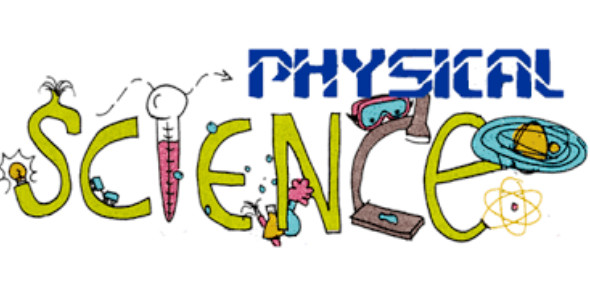Physical Science Practice Quiz: Trivia

15 Questions | Total Attempts: 562SettingsPhysical science practice quiz trivia. The distance one takes on the speed they choose and the time taken to get from one point to another. One way to solve the solution that includes two of these to find one is through the use of graphs. Take up the quiz and get to do see how much you know about reading said graphs and the relationship between speed, time and distance.

• 1.
The type of relationship represented between variables in the graph pictured is:
• A.

Strong relationship

• B.

Weak relationship

• C.

Inverse relationship

• D.

No relationship

• 2.
When constructing a graph, the x-axis is most closely related to:
• A.

Dependent variable

• B.

Vertical axis

• C.

Independent variable

• D.

The slope

• 3.
On this graph, the dependent variable is:
• A.

Distance

• B.

Speed

• C.

Acceleration

• D.

The slope of the speed vs distance curve

• 4.
Use the graph to predict the speed of the car when the car is at 60 cm
• A.

320cm/s

• B.

130cm/s

• C.

200cm/s

• D.

250cm/s

• 5.
The slope of a distance vs. time graph is equal to:
• A.

Distance

• B.

Speed

• C.

Acceleration

• D.

None of the above

• 6.
Calculate the speed of the object from the position vs. time graph shown below
• A.

0.5m/s

• B.

0.75m/s

• C.

2m/s

• D.

3m/s

• 7.
Match the position vs. time graph with the correct speed vs. time graph.
• A.

A

• B.

B

• C.

C

• D.

D

• 8.
At which of the following points on the graph is the speed the greatest?
• A.

1

• B.

2

• C.

3

• D.

4

• 9.
A bicycle rider would NOT be accelerating if her:
• A.

Direction and speed were unchanged.

• B.

Direction changed and speed was constant.

• C.

Speed increased and direction was unchanged.

• D.

Speed decreased.

• 10.
A rocket sled accelerates from 10 m/sec to 50 m/sec in 2 seconds. What is the acceleration ofthe sled?
• A.

10m/s/s

• B.

20m/s/s

• C.

25m/s/s

• D.

40m/s/s

• 11.
In this speed vs. time graph, the object at point C
• A.

Speeding up

• B.

Acceleration = 0

• C.

Slowing down

• D.

Not moving

• 12.
The rate of change in the velocity of an object is called ____________________.
• A.

Distance

• B.

Time

• C.

Acceleration

• D.

Speed

• 13.
The speed you have at a specific point in your journey is best called ____________________ speed.
• A.

Instantaneous

• B.

Average

• C.

Acceleration

• D.

Fastest

• 14.
A snail can move approximately 0.50 meters per minute. How many meters can snail travel in 15 minutes?
• A.

30m

• B.

0.02m

• C.

20m

• D.

7.5m

• 15.
A parachute on a dragster racing-car opens and changes the speed of the car from 85 m/sec to 0 m/sec in a period of 4.5 seconds. What is the acceleration of the car?
• A.

382.5m/s/s

• B.

18.9m/s/s

• C.

12m/s/s

• D.

150m/s/s

Related TopicsBack to top